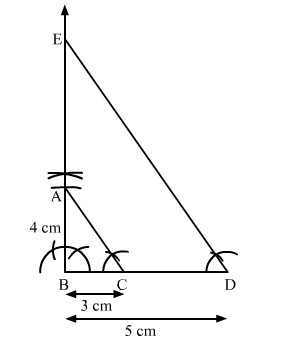# Draw a right triangle in which sides (other than hypotenuse) are of lengths 4 cm and 3 cm.`
Question:

Draw a right triangle in which sides (other than hypotenuse) are of lengths $4 \mathrm{~cm}$ and $3 \mathrm{~cm}$. Then, construct another triangle whose sides are $\frac{5}{3}$ times the corresponding sides

of the given triangle.

Solution:

Steps of Construction

Step 1. Draw a line segment BC = 3 cm.

Step 2. At B, draw ">XBC = 90º.

Step 3. With B as centre and radius 4 cm, draw an arc cutting BX at A.

Step 4. Join AC. Thus, a right ∆ABC is obtained.

Step 5. Extend BC to D such that BD $=\frac{5}{3} B C=\frac{5}{3} \times 3 \mathrm{~cm}=5 \mathrm{~cm}$.

Step 6. Draw DE || CA, cutting BX in E.Here, $\triangle \mathrm{BDE}$ is the required triangle similar to $\triangle \mathrm{ABC}$ such that each side of $\Delta \mathrm{BDE}$ is $\frac{5}{3}$ times the corresponding side of $\triangle \mathrm{ABC}$.